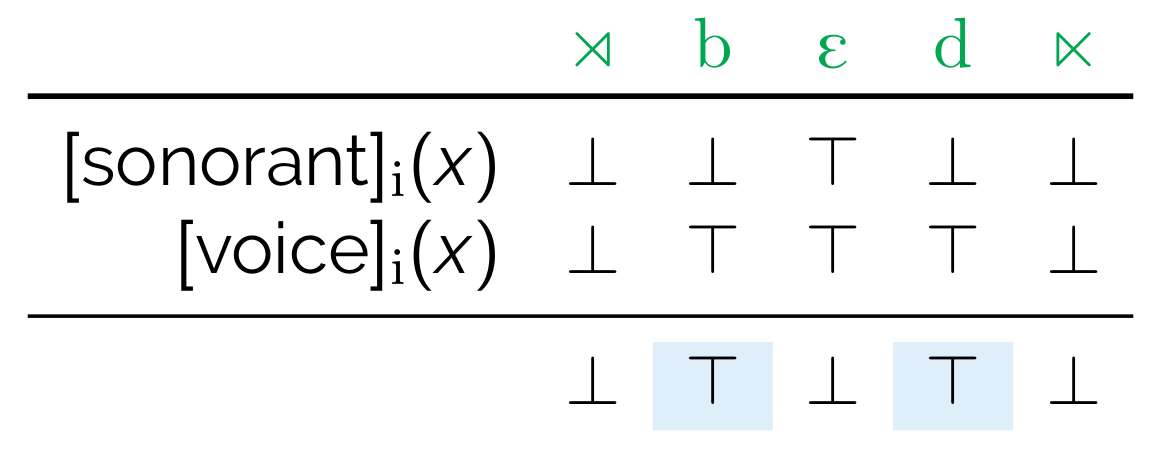# Logical language

The core of BMRS syntax are expressions of the form

$\mathtt{if}~A~\mathtt{then}~B~\mathtt{else}~C$

that return boolean values ($$\top$$ or $$\bot$$)

For example,

$\mathtt{if}~[\mathrm{son}]_i(x)~\mathtt{then}~\bot~\mathtt{else}~[\mathrm{voi}]_i(x)$

...is $$\top$$ iff $$x$$ is a voiced obstruent, as shown below.Furthermore, $$A$$, $$B$$, or $$C$$ can be another expression. Usually this is $$C$$, to chain together expressions, as in

$\begin{array}[t]{l} \mathtt{if}~[\mathrm{son}]_i(x)~\mathtt{then}~\bot~\mathtt{else}~\\ \mathtt{if}~[\mathrm{voi}]_i(x)~\mathtt{then}~\top~\mathtt{else}~\bot \end{array}$

As is perhaps obvious at this point, expressions define properties. For example, we can explicitly define what it means to be a voiced obstruent using the expression above.

$\left[\begin{array}{l}-\mathrm{son}\\+\mathrm{voi}\end{array}\right]_i(x):=\mathtt{if}~[\mathrm{son}]_i(x)~\mathtt{then}~\bot~\mathtt{else}~[\mathrm{voi]_i(x)}$

Or what it means to be word-final.

$\mathrm{final}_i(x):=\mathtt{if}~\ltimes(s(x))~\mathtt{then}~\top~\mathtt{else}~\bot$

And then what it means to be a word-final voiced obstruent.

$\mathrm{D\#}_i(x):=\mathtt{if}~\left[\begin{array}{l}-\mathrm{son}\\+\mathrm{voi}\end{array}\right]_i(x)~\mathtt{then}~\mathrm{final}(x)~\mathtt{else}~\bot$